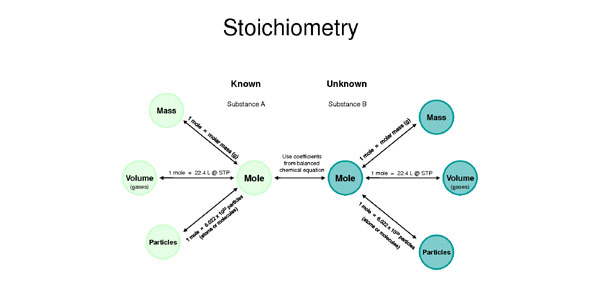# Stoich Quiz Pt 2

12 Questions | Attempts: 479
ShareSettingsFor chemical reactions to occur we need to have two or more elements being combined depending on the expected end chemical product. The quiz below is on the measure of the relative quantities of the elements. Enjoy.

• 1.
How many moles of H2O will be produced from the complete combustion of 2.4 grams of CH4? CH4 + 2O2 ® CO2 + 2H2O
• A.

0.15

• B.

0.30

• C.

1.5

• D.

3.0

• E.

4.5

• 2.
If 25.0 g of each reactant were used in performing the following reaction, which would be the limiting reactant?      3PbO2 + Cr2(SO4)3 + K2SO4 + H2O ® 3PbSO4 + K2Cr2O7 + H2SO4
• A.

PbO2

• B.

H2O

• C.

K2SO4

• D.

PbSO4

• E.

Cr2(SO4)3

• 3.
If you have 4.5 moles of lead(IV) nitrate howmay moles of silver nitrate can be produced according to the following reaction? Pb(NO3)4 + 4AgCl ---> 4AgNO3 + PbCl4
• A.

1.2n

• B.

4.5n

• C.

16n

• D.

18n

• 4.
Starting with 31g of Al in the following reaction will produce hao many grams of Na? Na3PO4 + Al ---> AlPO4 + 3Na
• A.

8.8g

• B.

9.0g

• C.

79g

• D.

79.2g

• 5.
If you counted 6.0 x 10^24 molecules of hydrogen gas how many molecules of oxygen gas will be need to completely react the amount according to the following equation? 2H2 + O2 ---> 2H2O
• A.

3.0 x 10^23

• B.

1.2 x 10^24

• C.

3.0 x 10^24

• D.

1.2 x 10^25

• 6.
40.0g of Sugar(C6H12O6) will product what volume of carbon dioxide according to the following equation? C6H12O6 + 6O2 ---> 6H2O + 6CO2
• A.

0.829L

• B.

0.830L

• C.

29.8L

• D.

29.9L

• 7.
Using the following equation how many liters of hydrogen gas will be needed to react with 5.0L of oxygen gas? 2H2 + O2 ---> 2H2O
• A.

2.5L

• B.

10L

• C.

10.L

• D.

10.0L

• 8.
Starting with 20.5L of hydrogen gas how many molecules of amonia can be made according to this equation? N2 + 3H2 ---> 2NH3
• A.

8.26 x 10^22

• B.

3.67 x 10^23

• C.

8.26 x 10^23

• D.

3.67 x 10^24

• 9.
If starting with 1.2 x 10^24 atoms of potassium how many grams of potassium oxide can you produce using the following equation? 4K + O2 ---> 2K2O
• A.

9.39g

• B.

9.4g

• C.

93.9g

• D.

94g

• 10.
3.50 x 10^25 compounds of calcium carbonate will produce what volume of carrbon dioxide according to the following equation? CaCO3 ---> CaO + CO2
• A.

13L

• B.

130L

• C.

1300L

• D.

13000L

• 11.
If 25g of NaCl is produced by the following reaction how many atoms of sodium would be required?2Na + Cl2 ---> 2NaCl
• A.

2.59 x 10^23

• B.

2.6 x 10^23

• C.

5.18 x10^23

• D.

5.19 x 10^23

• 12.
When reacting 448L of hydrogen gas with oxygen gas how many grams of water can be produced?2H2 + O2 ---> 2H2O
• A.

360 g

• B.

361 g

• C.

362 g

• D.

367 g

## Related TopicsBack to top
×

Wait!
Here's an interesting quiz for you.